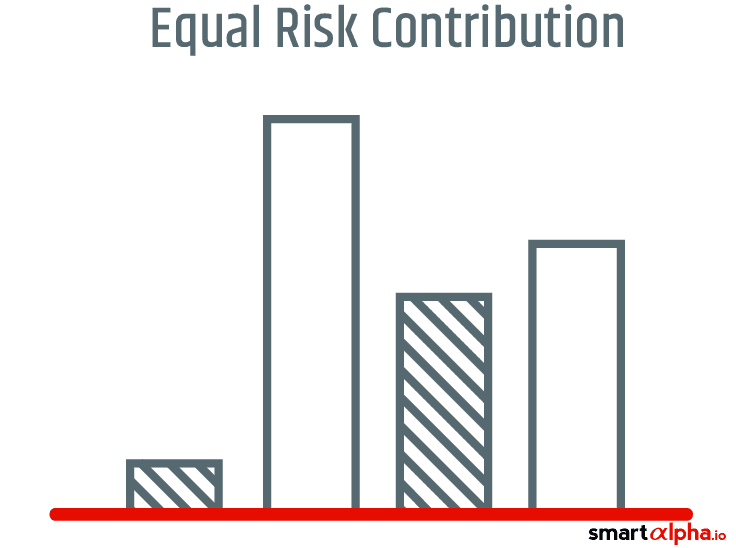# Equal Risk Contribution IndicesEqual risk contribution indices weight stocks in such a way that they contribute equally to the risk of a portfolio. This relatively new smart beta investment strategy aims to control volatility risk, and in doing so, improve returns on a risk-adjusted basis. Greater risk is associated with a higher risk premium.

The equal risk model is a type of risk-parity strategy, but with a slightly different slant. Risk parity infers that individual investments within a fund have the same level of volatility, while an equal risk approach engineers constituents’ weightings so that they contribute equal levels of volatility.

Indices which are built on equal risk terms use inverse volatility weightings – in other words, the more volatile or risky a stock, the lower its weighting in a portfolio, and vice versa. In addition, this approach can take into account the correlation of constituents, where a low correlation to the overall portfolio results in a greater weighting.

The idea is to diversify a fund and reduce the concentration of risk towards sectors, asset classes or other factors. In short, these strategies target less volatile returns relative to traditional indices which weight stocks by their size.

Sector weights are sometimes capped at predefined levels to avoid unintended over-concentration, which would effectively dilute the risk-smoothing benefits of this strategy. Meanwhile, concentration towards a specific risk is automatically tempered when its volatility and or correlation rises, since its weighting in the portfolio reduces.

The equal risk contribution strategy is in some ways similar to the minimum variance approach, which gives greater weighting to low volatility, stable stocks in order to control volatility and risk.

But unlike a number of other volatility-reducing strategies, compiling an equal risk index does not require analysis or predictions of future returns. As such, equal risk approaches would likely suit scenarios in which the inherent and future risks of different strategies or assets are relatively clear, but future returns are less predictable.

Given current market conditions – marred by political and financial uncertainty, equal risk contribution indices have flourished in number and in popularity as investors recognise the value of effective risk management.

As a smart beta strategy, equal risk contribution assigns alternative weightings to indices in an effort to attain desired outcomes – in this case, volatility control. The performance of smart beta strategies is measured against benchmark indices on a risk-adjusted basis.

While equal risk contribution models may favour risk control over maximising returns, many other smart beta investment strategies aim to generate superior returns relative to the benchmark by targeting specific risks. Among these are value and momentum strategies.

These alpha strategies can be implemented through long-only positions (the approach usually taken by a mutual fund or smart beta portfolio), or long-short trades that target absolute returns (the approach taken by risk premia funds and hedge funds).

Alpha strategies strive to capture the risk premium attached to financial assets which are seen as riskier than the market.

Equal risk product launches

FTSE launched its Global Equal Risk Contribution Index Series in the second half of 2013. The constituents of the indices correspond to the underlying All-World Index Series, which is reviewed twice a year.

FTSE said at the time of its series launch that its equal risk contribution alternative had produced similar returns to its traditional size-based series, but with much lower volatility.

A number of other index providers offer equal risk weighted indices, including major providers like S&P Dow Jones Indices and Stoxx.

The EURO iSTOXX 50 Equal Risk Index, for example, is based on the EURO STOXX 50 Index, shifting from a free-float size weighting approach to equal risk, where allocations are reviewed as regularly as each month. This index does not include a cap factor.

The S&P GSCI Risk Weight index tracks the traditional commodities index on risk-weighted terms. Each commodity sector contributes a risk weighting based on its volatility and correlation with the other commodity types. The standard index on which it is based, the S&P GSCI, is weighted by market production figures.

These smart beta indices differ from alpha-orientated strategies such as value and momentum strategies.